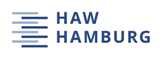# Selected Statistics in Aircraft Design

Author: Lucie Morichon

Project

## Abstract

Trends and relationships have been extracted from aircraft design literature in order to comment on 10 relationships between aircraft parameters. Statistical research has been undertaken.

A data base was created from 53 passengers aircraft. Thanks to selected data and literature excerpts, trends have been discussed and relationships have been statistically analyzed using regression tools. Each time a regression curve was acceptable, an equation was written and interpreted. For one equation corresponding to literature, a coefficient could be optimized. For newly found equations, interpretation was only done after validation of the underlying physical reality. Sometimes, equations were mathematically feasible but had no physical meaning. These equations had to be discarded. In two cases, no relationship or trend was revealed by analysis. Many factors can be responsible for the failure of finding a correlation. A regression may simply not exist at all.

In a nutshell, this project confirms relationships among aircraft parameters from literature and tries to optimize related equations. The project created new equations which were up to now not available in literature or questions the validity of equations from literature.